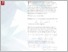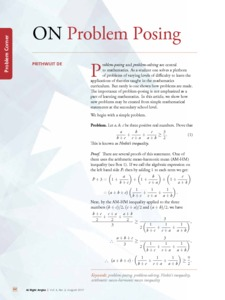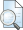# On problem posing

De, Prithwijit (2017) On problem posing. At Right Angles, 6 (2). pp. 82-85. ISSN 2582-1873Preview
Text - Published Version

## Abstract

Problem-posing and problem-solving are central to mathematics. As a student one solves a plethora of problems of varying levels of difficulty to learn the applications of theories taught in the mathematics curriculum. But rarely is one shown how problems are made. The importance of problem-posing is not emphasized as a part of learning mathematics. In this article, we show how new problems may be created from simple mathematical statements at the secondary school level. We begin with a simple problem. Problem. Let a, b, c be three positive real numbers. Prove that a b c 3 + + ≥ . (1) b + c c + a a + b 2 This is known as Nesbit’s inequality. Proof. There are several proofs of this statement. One of them uses the arithmetic mean-harmonic mean (AM-HM) inequality (see Box 1). If we call the algebraic expression on the left hand side P, then by adding 1 to each term we get:

Item Type: Articles in APF Magazines
Authors: De, Prithwijit
Document Language:
Language
English
Uncontrolled Keywords: Problem-posing, Problem-solving, Nesbit's inequality, Arithmetic mean-harmonic mean inequality
Subjects: Natural Sciences > Mathematics
Divisions: Azim Premji University > University Publications > At Right Angles
Full Text Status: Public
URI: http://publications.azimpremjiuniversity.edu.in/id/eprint/1373
Publisher URL: http://apfstatic.s3.ap-south-1.amazonaws.com/s3fs-...View Item